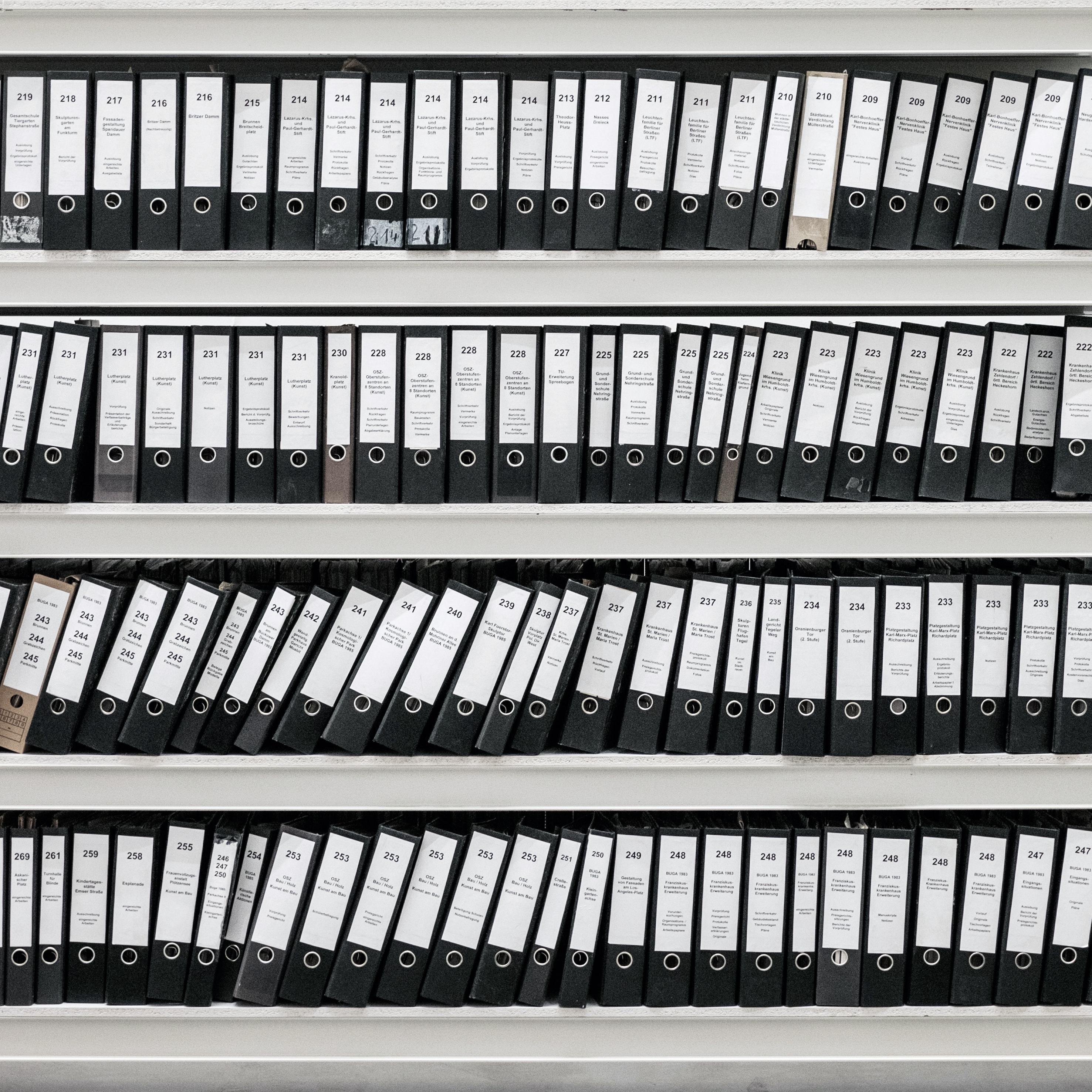# Why Most Files Can't Be CompressedThis is an assumption proof given in the Cardiff Uni Maths Coding Theory and Data Compression Course. It makes sense if you understand what we mean by “most files.”; i.e. literally any random string of data.

So, why, in most cases, can’t any old file be compressed? Lets start off at the beginning obvious: let $$A,B$$ be files, and they get compressed to $$C,D$$ respectively. Now $$C=D$$ if and only if $$A=B$$. So every compression must be unique if its source is unique.

Now, we can look into what happens when we have every possible combination of a file length.

 Let $$A =n$$ then once compressed it removes 10% of the redundant data, or $$C =0.9n$$.

For any file with length $$n$$ we have $$2^{n}$$ possible files (for every ordering of data) each of which would need a compressed equivalent.

The number of file sizes up to $$2^{0.9n}$$ is:

This ratio of #of files to #of compressed possibilities: $$2^{0.9n +1}$$/$$2^n$$ tends to 0 as $$n \rightarrow \infty$$

The concept of Data compression means to reduce redundant data in a file, however most of the $$2^n$$ possibilities are random and have no redundant data. Data compression will only work on files where there is a possibility to remove redundancy that exists.